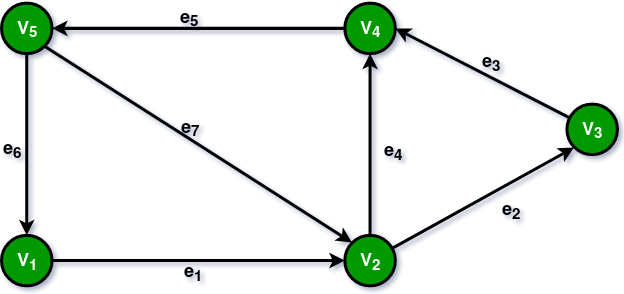Open in App
Not now

# Outdegree of a Graph

• Last Updated : 17 Mar, 2023

Outdegree of a vertex is defined as the number of outgoing edges from a vertex in a directed graph.

### Significance of Outdegree:

• The outdegree of a directed graph vertex, which reflects the total number of edges emanating from that node, is always positive and never negative.
• If a directed graph’s vertex does not have any edges leading to other vertices, then its outdegree will be 0.
• The total number of edges in a graph is equal to the sum of all outdegrees because in a directed graph, there is precisely one vertex at each end of each edge.
• Vertices with an outdegree of zero are known as sink vertices.

### How to calculate Outdegree of a Node?Example of a directed graph

Consider the above directed graph. To determine a vertex’s outdegree in a directed graph, one must count the number of directed edges that leave from that vertex.

How to determine a vertex’s outdegree in a directed graph is as follows:

• Choose to pick the vertices whose outdegrees you wish to know about.
• Check how many outgoing directed edges there are from that vertex by going along the edges of the graph.
• Keep track of how many directed edges in total come from that node.
• The vertex’s outdegree is equal to this number.

In the above graph, there is only one outgoing edge from the vertex (V1) i.e. edge e1. Hence the outdegree of the vertex (V1) is 1. Similarly,

• Outdegree (V2) = 2 as there are two outgoing edges e2 and e4.
• Outdegree (V3) = 1 as there is only one outgoing edge e3.
• Outdegree (V4) = 1 as there is only one outgoing edge e5.
• Outdegree (V5) = 2 as there are two outgoing edges e6 and e7.

### What else can you read?

My Personal Notes arrow_drop_up
Related Articles